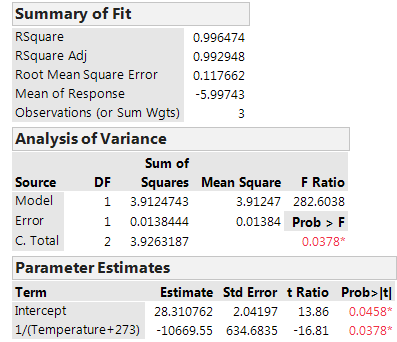BookmarkSubscribe
Choose Language Hide Translation Bar

Fitting a line taking into account different error bars on data points

Hi, I wish to get the gradient and standard error of gradient of a straight line being fitted between three data points. Ordinarily this would be a very simple bit of linear regression. However, the three data points each have a standard error associated with them, as each point is in itself the natural log of the gradient of another linear regression fit. If I fit a straight line to my three data points in the normal way, the standard error of the gradient will be vastly underestimated, because it fails to take into account the errors associated with the data points.

Here are some visualisations of the data to explain a bit more:

1) Fitting response as a function of time, grouped by temperature.2) Fitting the logs of (-1 x 3 gradients above) as a function of (1/temperature). The important bit I need is the gradient and standard error of gradient of this graph...Any thoughts on how I might go about preventing myself from underestimating the standard error of the gradient in part 2) please?